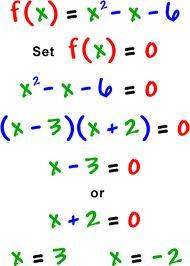Polynomials and Factoring

This quiz will test your knowledge of polynomials and factoring trinomials.

published on July 24, 20123 responses 0 5.0★ / 5

Which is the name of the following polynomial? 6x

Constant Monomial
Linear Monomial
Linear Binomial
None of the above

Which of the following are polynomials? Exponents are written by using this symbol ^ followed by the exponent value. Ex, x^3

Select the three correct answers
6
5x^2+2y
1
0

11x^2+3x-1
11x^3+2x-3
11x^4+5x-4
11x5+6x-5

(m-6)(m-6)
(m-9)(m+10)
(m+9)(n+10)
(m+10)(m+8)

(x+2)(x-6)
(x+5)(x-6)
Not factorable.

(3p-5)(p+1)
(3p-5)(p-1)
(3p-5)(q+1)
Not Factorable

(4n+3)(4n-3)
(4n+4)(4n-4)
Not factorable.

(8r^2+1)(r-8)
(8r^5-1)(r-2)
I'm lost.
Not Factorable.

2x^4+9x
6x^8-6x
6x^4-6x